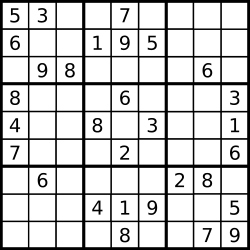## Valid Sudoku

06/26/2016 Hash Table

## Question

Determine if a Sudoku is valid, according to: Sudoku Puzzles - The Rules.

The Sudoku board could be partially filled, where empty cells are filled with the character '.'.Note:

A valid Sudoku board (partially filled) is not necessarily solvable. Only the filled cells need to be validated.

## Solution

Result: Accepted Time: 4ms

Here should be some explanations.

bool isValidSudoku(char** board, int boardRowSize, int boardColSize) {
int mat, col, row;
int i,j,x,y,tmp;
for(i = 0; i < boardRowSize; i++)
{
for(j = 0; j < 10; j++)
mat[j]=row[j]=col[j]=0;
for(j=0;j<boardColSize;j++)
{
x = (i/3)*3 + j/3;
y = (i%3)*3 + j%3;
if(board[i][j]!='.')
{
tmp = board[i][j] -'0';
if(row[tmp])
return false;
row[tmp]=1;
}
if(board[j][i]!='.')
{
tmp = board[j][i] -'0';
if(col[tmp])
return false;
col[tmp] = 1;

}
if(board[x][y]!='.')
{
tmp = board[x][y] - '0';
if(mat[tmp])
return false;
mat[tmp] = 1;
}
}
}
return true;
}


Complexity Analytics

• Time Complexity: $O(1)$
• Space Complexity: $O(1)$